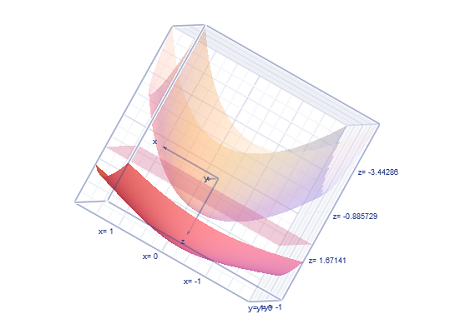# Find the volume of the solid below the hyperboloid { z = 5 - \sqrt{(1+x^2+y^2)} } and above the...

## Question:

Find the volume of the solid below the hyperboloid {eq}z = 5 - \sqrt{(1+x^2+y^2)} {/eq} and above the following region the solid bounded by the paraboloid {eq}z= 2- x^2-y^2 {/eq} and the plane z=1

## Definite Integrals:

When there is a definite integral with some limits in the x, y and z variables, then it means that integral is for the volume calculation. The order of the integral is the important thing that points to the equation of the curves that bounds the volume.

The three curves are plotted below:here the volume integral is set up with the order dz dy dx

where z varies from:

{eq}z= 2- x^2-y^2 {/eq}

to

{eq}z = 5 - \sqrt{(1+x^2+y^2)} {/eq}

And the equation is x and y is:

{eq}1= 2- x^2-y^2\\ => x^2+y^2=1\\ {/eq}

So y varies from:

{eq}y=-\sqrt{1-x^2} {/eq}

to

{eq}y=\sqrt{1-x^2} {/eq}

Finally, x varies from:

x=-1 to x=1

So the volume integral is:

{eq}\int_{-1}^{1}\int_{-\sqrt{1-x^2}}^{\sqrt{1-x^2}}\int_{ 2- x^2-y^2}^{5 - \sqrt{(1+x^2+y^2)}} dz dy dx\\ {/eq}

Now the final volume is the final value of the integral, as follows:

{eq}\int _{\:2-\:x^2-y^2}^{5\:-\:\sqrt{\left(1+x^2+y^2\right)}}\:dz\\ =\left[z\right]^{5-\sqrt{1+x^2+y^2}}_{2-x^2-y^2}\\ =x^2+y^2+3-\sqrt{x^2+y^2+1}\\ {/eq}

The integral that is left is;

{eq}\int _{-1}^1\int _{-\sqrt{1-x^2}}^{\sqrt{1-x^2}}x^2+y^2+3-\sqrt{x^2+y^2+1}\:dy\:dx\\ {/eq}

Converting to the polar form is;

{eq}-1\le \:x\le \:1\\ => 0\le \:r\le \:1\\ =>0\le \:\theta \le \:2\pi \\ {/eq}

So the polar double integral that we get is;

{eq}\int _0^{2\pi }\int _0^1\left(r^2+3-\sqrt{r^2+1}\right)rdrd\theta \\ {/eq}

Now the integral:

{eq}\int _0^1\left(r^2+3-\sqrt{r^2+1}\right)rdr\\ =\int _0^1r^3dr+\int _0^13rdr-\int _0^1r\sqrt{r^2+1}dr\\ =\left[\frac{r^4}{4}\right]^1_0+3\left[\frac{r^2}{2}\right]^1_0-\left[\frac{(\sqrt{r^2+1})^3}{3}\right]^1_0 \\ =\frac{25}{12}-\frac{2\sqrt{2}}{3}\\ {/eq}

Now the outer most integral is;

{eq}\int _0^{2\pi }\left(\frac{25}{12}-\frac{2\sqrt{2}}{3}\right)d\theta \\ =\left[\frac{25-8\sqrt{2}}{12}\theta \right]^{2\pi }_0\\ =\frac{\pi \left(25-8\sqrt{2}\right)}{6} {/eq}

So this gives the final volume.